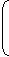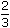# Aptitude - True Discount - Discussion

### Discussion :: True Discount - General Questions (Q.No.6)

6.

Goods were bought for Rs. 600 and sold the same for Rs. 688.50 at a credit of 9 months and thus gaining 2% The rate of interest per annum is:

[A].
 16 2 % 3
[B].
 14 1 % 2
[C].
 13 1 % 3
[D]. 15%

Explanation:

 S.P. = 102% of Rs. 600 =102 x 600= Rs. 612. 100

Now, P.W. = Rs. 612 and sum = Rs. 688.50.T.D. = Rs. (688.50 - 612) = Rs. 76.50.

Thus, S.I. on Rs. 612 for 9 months is Rs. 76.50.Rate =100 x 76.50% = 16%
 612 x 3 4

 Aravind said: (Dec 9, 2010) SP = 102 % of 600 cannot be rs. 612 Hence the answer is wrong please correct the error Regards Aravind

 Omer said: (Jul 14, 2011) How to put 9.375 in fraction in easy method to get 16*2/3 Kindly help me out

 Abbin said: (Aug 9, 2011) On reading the Q, I had a confusion that the 2% is for Rs.600 or Rs.688.50. I think that also should be mentioned in the question.

 Sirisha said: (Sep 30, 2011) As the gain is 2% then gain in Rupees is calculated as gain=(2/100)*cost price =(2/100)*600 =12 So the selling price that is the PW value becomes 600+12=612 Then TD=amount-PW=688.50-612=76.50 from this we claculate R using the formula P.W=(100*T.D)/(R*T)

 Mukesh Vyas said: (Apr 18, 2012) Please resolve value 102%.

 Jyothi said: (Apr 3, 2013) Purchase price = 600 consider it 100%. Gain 2% on purchase price. Present value of selling price = 100% + 2% = 102%.

 Anjali Singh said: (Oct 15, 2013) Unable to understand how we get 102%of 600 = 612. What is the formula behind it?

 Likhitha said: (Jun 20, 2014) @Anjali. (102/100)*600 = 612. But I can't understand why? P.W = (amount*100)/(R*T) this formula was used as real one is P.W = (amount*100)/(100+(R*T).

 Vignesh said: (Sep 25, 2014) What is pw ?

 Aparna said: (Jul 8, 2015) Shall I know what is the formula for are how that 76.5 came here in the formula of rate?

 Md. Kamrujjaman said: (May 9, 2016) Here gaining 2% means the gain in purchase price not 2% interest for 9 months. So the purchase price + gain (profit) = sale price. = 600 + 600 * 2% = 612. Now discount 688.50 for 9 months taking 612 as P. W. (present worth). For example: 612 = (688.50 * 100)/[100 + (x * 9/12)]. X = 50/3=>rate of interest per annum.

 Venkatesh said: (May 19, 2016) I'm little confused. Is there any easy methods to solve and understand this problem?

 Srk said: (Jun 21, 2016) 2% profit, hence, 600*2/100 = 12 Total = 612 So, net price 688.50 - 612 = 76.50 And the formula is for rate is used.

 Deepa A J said: (Jan 11, 2017) Why 3/4 is taken in the problem?

 Anchal said: (Feb 1, 2017) @Kamrujjaman. 9/12 =3/4.

 Sushmita said: (Mar 30, 2017) Cost price= 600. He sold the goods at a credit of 9 months, it means that the buyer will pay him after 9 months rupees 688.50. It's also given in the question that the seller has 2% profit. So the Present value (PV) of 688.50 should be equal to 102/100 * 600 = 612. It means SI on 612 for 9 months = 688.50 - 612 = 76.50. 612*R*9/100 * 12 = 76.50 Solving we get R = 16.666% = option A. Hope it's clear enough.

 Vijay said: (Jun 21, 2018) Well, it's simple. Goods were bought for 600. Sold for 688.50 at the credit of 9 months. Gain = 2%, Rate = x%. P.W = C.P + Gain. = 600 + 2% of 600, = 600 + 12 = 612. Simple Interest = 688.50 - 612 = 76.50, By formula, P.W * R * T /100 = S.I. R = S.I * 100 / (P.W * T). R = 76.50 * 100 / (612 * 9/12). R = 16.66%.

 Kiran Roy said: (Oct 8, 2018) Why it is 9/12 instead of 12/9?

 Sowmya said: (Dec 21, 2018) What is the formula for rate of interest?

 Dulle said: (May 3, 2020) @Sowmya The formula of Interest = PRT/100.